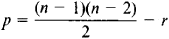# Genus of a Curve

The following article is from The Great Soviet Encyclopedia (1979). It might be outdated or ideologically biased.

## Genus of a Curve

a number characterizing an algebraic curve. The genus of the nth degree curve f(x, y)= 0 iswhere r is the number of double points. When more complex singular points are present, they are counted as the corresponding number of double points; for example, a cusp is counted as one double point, and a triple point is counted as two.

Second-degree curves are of genus 0. Third-degree curves can be of genus 0 or 1. For example, yx3= 0 is of genus 1. On the other hand, the semicubical parabola y2 – x3 = 0, which has one cusp, is of genus 0, as is the folium of Descartes x3 + y3 – 3axy = 0, which has one double point. Curves of genus 0 are called unicursal curves.